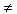Introduction

A function is considered to be a quadratic function if the equation has a degree or highest exponent of 2. The standard form of a quadratic equation is y = ax2 + bx + c where a, b, and c are real numbers and a0. All functions of this form have similar characteristics.

Listen